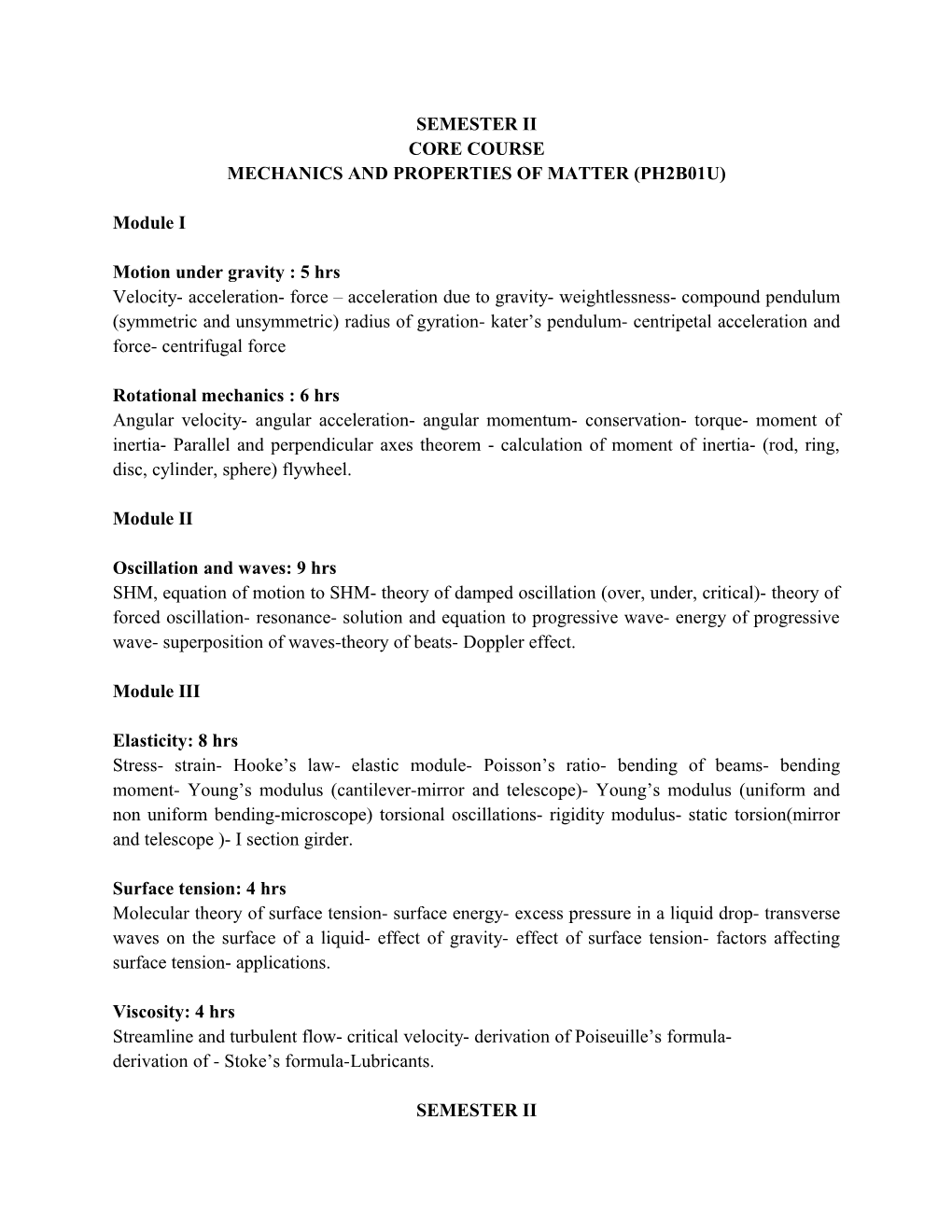# Mechanics and Properties of Matter (Ph2b01u)SEMESTER II

CORE COURSE

MECHANICS AND PROPERTIES OF MATTER (PH2B01U)

Module I

Motion under gravity : 5 hrs

Velocity- acceleration- force – acceleration due to gravity- weightlessness- compound pendulum (symmetric and unsymmetric) radius of gyration- kater’s pendulum- centripetal acceleration and force- centrifugal force

Rotational mechanics : 6 hrs

Angular velocity- angular acceleration- angular momentum- conservation- torque- moment of inertia- Parallel and perpendicular axes theorem - calculation of moment of inertia- (rod, ring, disc, cylinder, sphere) flywheel.

Module II

Oscillation and waves: 9 hrs

SHM, equation of motion to SHM- theory of damped oscillation (over, under,critical)- theory of forced oscillation- resonance- solution and equation to progressive wave- energy of progressive wave- superposition of waves-theory of beats- Doppler effect.

Module III

Elasticity: 8 hrs

Stress- strain- Hooke’s law- elastic module- Poisson’s ratio- bending of beams- bending moment- Young’s modulus (cantilever-mirror and telescope)- Young’s modulus (uniform and non uniform bending-microscope) torsional oscillations- rigidity modulus- static torsion(mirror and telescope )- I section girder.

Surface tension: 4 hrs

Molecular theory of surface tension- surface energy- excess pressure in a liquid drop- transverse waves on the surface of a liquid- effect of gravity- effect of surface tension- factors affecting surface tension- applications.

Viscosity: 4 hrs

Streamline and turbulent flow- critical velocity- derivation of Poiseuille’s formula-

derivation of - Stoke’s formula-Lubricants.

SEMESTER II

CORE COURSE PRACTICAL

MECHANICS AND PROPERTIES OF MATTER (PH2B01U)

1. Cantilever- pin & microscope –Determination of Young’s modulus

2. Carey Foster’s Bridge-Measurement of resistivity

3. Symmetric Compound Pendulum-Determination of radius of gyration(K) andAcceleration due to gravity (g)

4. Surface tension - Capillary rise method

5. Half wave rectifier with and without filter-ripple factor and load regulation

6. Conversion of Galvanometer into voltmeter

7. Viscosity-constant pressure head- coefficient of viscosity (η) of the liquid

8. Spectrometer- Refractive Index of material of Prism

9. Field along the axis of a coil-Variation of magnetic field along the axis of a circular coil

10. Electro chemical equivalent of copper

SEMESTER II

COMPLEMENTARY COURSE: PHYSICS FOR MATHEMATICS

ELECTRIC AND MAGNETIC PHENOMENA, THERMODYNAMICS AND SPECIAL THEORY OF RELATIVITY (MT2C01U)

Module I

Dielectric materials (7 hrs)

Dielectrics- polar and non-polar dielectrics- polarization- sources of polarization- Gauss’s law in dielectrics- permittivity- dielectric displacement vector- dielectric constant-susceptibility- ferroelectricity

Magnetic Materials (7 hrs)

Magnetization in materials- linear and non-linear materials- diamagnetism-paramagnetism-ferromagnetism- hysteresis- ferromagnetic domains- antiferromagnetism- ferrimagnetism

Module II

Thermodynamics (12 hrs)

Thermodynamic systems- thermodynamic equilibrium- thermodynamicprocesses- isothermal process- adiabatic process- zeroth law of thermodynamics- first law of thermodynamics- heat engine- the Carnot engine- refrigerator- concept of entropy- second law of thermodynamics- third law of thermodynamics- Maxwell’s thermodynamic relations

Module III

Special theory of relativity (10 hrs)

Introduction- Galilean transformation- Newtonian principle of relativity- special theory- postulates- Lorentz transformation- length contraction- time dilation- relativity of simultaneity- addition of velocities- relativistic mass transformation- mass energy relation

SEMESTER II

COMPLEMENTARY COURSE: PHYSICS FOR MATHEMATICS PRACTICAL

ELECTRIC AND MAGNETIC PHENOMENA, THERMODYNAMICS AND SPECIAL THEORY OF RELATIVITY (MT2C01)

1. Cantilever – Scale and Telescope-Determination of Young’s modulus

2. Asymmetric Compound Pendulum-Determination of K and g

3. Coefficient of Viscosity – Constant pressure head

4. Spectrometer - Refractive Index of material of prism.

5. Liquid lens - Refractive Index of glass using liquid of known refractive index

6. Potentiometer-Calibration of low range voltmeter

7. Characteristics of Zener diode

8. Construction of half wave rectifier with and without filter – Ripple factor andLoad regulation

9. Mirror Galvanometer – Figure of merit

10. Torsion pendulum - Rigidity modulus

SEMESTER II

COMPLEMENTARY COURSE: PHYSICS FOR CHEMISTRY

ELECTRIC AND MAGNETIC PHENOMENA, THERMODYNAMICS AND ELEMENTARY SOLID STATE PHYSICS (CH2C01U)

Module I

Dielectric materials (7 hrs)

Dielectrics- polar and non-polar dielectrics- polarization- sources of polarization- Gauss’s law in dielectrics- permittivity- dielectric displacement vector- dielectricconstant-susceptibility- ferro electricity

Magnetic materials (7 hrs)

Magnetization in materials- linear and non-linear materials- diamagnetism- paramagnetism-ferromagnetism- hysteresis- ferromagnetic domains- antiferromagnetism- ferrimagnetism

Module II

Crystalline solids (10 hrs)

Crystalline and amorphous solids- crystal lattice- basis- unit cell- lattice parameters- crystal systems- crystal planes and directions- miller indices- simple cubic- fcc -bcc hcp structures- packing fraction- NaCl structure- crystaldiffraction- Bragg’s law

Module III

Thermodynamics (12 hrs)

Thermodynamic systems- thermodynamic equilibrium- thermodynamic processes- isothermal process- adiabatic process- zeroth law of thermodynamics- first law of thermodynamics- heat engine- the Carnot engine- refrigerator- concept of entropy- second law of thermodynamics- third law of thermodynamics- Maxwell’s thermodynamic relations

SEMESTER II

COMPLEMENTARY COURSE: PHYSICS FOR CHEMISTRY PRACTICAL

ELECTRIC AND MAGNETIC PHENOMENA, THERMODYNAMICS AND ELEMENTARY SOLID STATE PHYSICS (CH2C01U)

SEMESTER II

1. Cantilever – Scale and Telescope-Determination of Young’s modulus

2. Asymmetric Compound Pendulum-Determination of K and g

3. Coefficient of Viscosity – Constant pressure head

4. Spectrometer - Refractive Index of material of prism.

5. Liquid lens - Refractive Index of glass using liquid of known refractive index

6. Potentiometer-Calibration of low range voltmeter

7. Characteristics of Zener diode

8. Construction of half wave rectifier with and without filter – Ripple factor and Load regulation

9. Mirror Galvanometer – Figure of merit

10. Torsion pendulum - Rigidity modulus